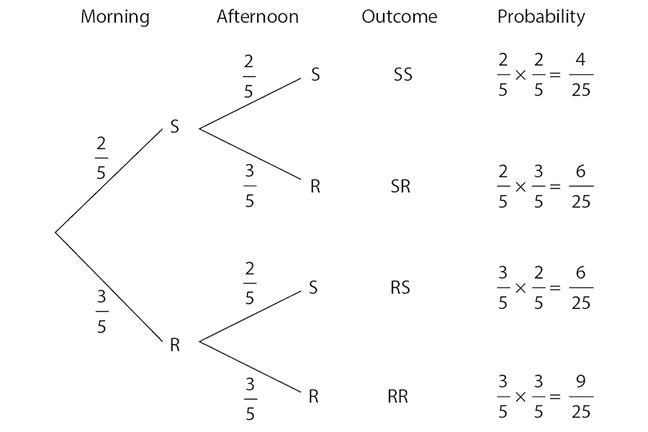## Want to keep learning?

This content is taken from the Cambridge University Press's online course, Teaching Probability. Join the course to learn more.
1.15

## Cambridge University PressProbability trees can be derived from expected frequency trees.

# From expected frequencies to probabilities

We do not start with probability trees, because they present students with a whole range of new concepts and techniques simultaneously, and many problems can be solved without them, using just frequencies.

Starting with the frequency tree and empirical data familiarises students with the tree structure. We then introduce the expected frequency tree, focusing on proportions to answer questions. In the weather experiment, we used a 25-day period, because the calculations of proportions for the morning and then the afternoon could be solved using only whole numbers. However, the choice of time period is arbitrary: in any experiment we need to specify how many trials there will be, but eventually we need to abstract the calculations from the experimental context so that we are not restricted to that number of trials.

On a frequency tree, the sum of the frequencies for the outcomes must be the same as the number of trials. The sum of the probabilities of the outcomes is 1, so in moving from the expected frequency tree to a probability tree, we have implicitly generalised the experiment. Instead of working with a number of trials, we agree that, whatever period might be chosen, it represents 100% of the trials. However, working with percentages on a probability tree should be avoided with students, because it is so easy to forget that a percentage is actually a fraction, not a whole number.

When moving from an expected frequency tree to a probability tree, we remove the boxes for results, and place the labels directly at the ends of the splits in the branches, as shown above. We also remove the arrows we put on the branches of the frequency tree, helping to emphasise that this is a different tree. The probabilities on the individual splits in the branches represent the number of times each event is expected as a fraction of the total number of trials represented by that split. The probability at the end of each entire branch represents the overall number of trials expected to result in that outcome as a fraction of the total number of trials.

Multiplying along the branches follows from the calculations that we did on the expected frequency tree. The proportion of sunny mornings was 40%, and the proportion of sunny mornings followed by sunny afternoons was 40% of 40%, which is the same as multiplying 2/5 by 2/5. If we want to know the probability of the event ‘a sunny afternoon’, then we need to add the probabilities for the relevant outcomes (i.e. for SS and RS) just as we would add frequencies on the expected frequency tree.

Do you agree that expected frequency trees are an important precursor to the more abstract idea of probability trees?